# The GLIMMIX Procedure

#### Graphics for LS-Mean Comparisons

The following subsections provide information about the ODS statistical graphics for least squares means produced by the GLIMMIX procedure. Mean plots display marginal or interaction means. The diffogram, control plot, and ANOM plot display least squares mean comparisons.

##### Mean Plots

The following SAS statements request a plot of the PressureTemp means in which the pressure trends are plotted for each temperature.

ods graphics on;
ods select CovParms Tests3 MeanPlot;
proc glimmix data=Yields;
class Vendor Pressure Temp;
model Yield = Pressure Temp Pressure*Temp;
random Vendor;
lsmeans Pressure*Temp / plot=mean(sliceby=Temp join);
run;
ods graphics off;


There is a significant effect of temperature and an interaction between pressure and temperature (Figure 45.24). Notice that the pressure main effect might be masked by the interaction. Because of the interaction, temperature comparisons depend on the pressure and vice versa. The mean plot option requests a display of the PressureTemp least squares means with separate trends for each temperature (Figure 45.25).

Figure 45.24: Tests for Fixed Effects

The GLIMMIX Procedure

Covariance Parameter Estimates
Cov Parm Estimate Standard
Error
Vendor 0.8602 0.7406
Residual 1.1039 0.3491

Type III Tests of Fixed Effects
Effect Num DF Den DF F Value Pr > F
Pressure 2 20 1.42 0.2646
Temp 1 20 6.48 0.0193
Pressure*Temp 2 20 3.82 0.0393

The interaction between the two effects is evident in the lack of parallelism in Figure 45.25. The masking of the pressure main effect can be explained by slopes of different sign for the two trends. Based on these results, inferences about the pressure effects are conducted for a specific temperature. For example, Figure 45.26 is produced by adding the following statement:

lsmeans pressure*temp / slicediff=temp slice=temp;


Figure 45.25: Interaction Plot for Pressure x Temperature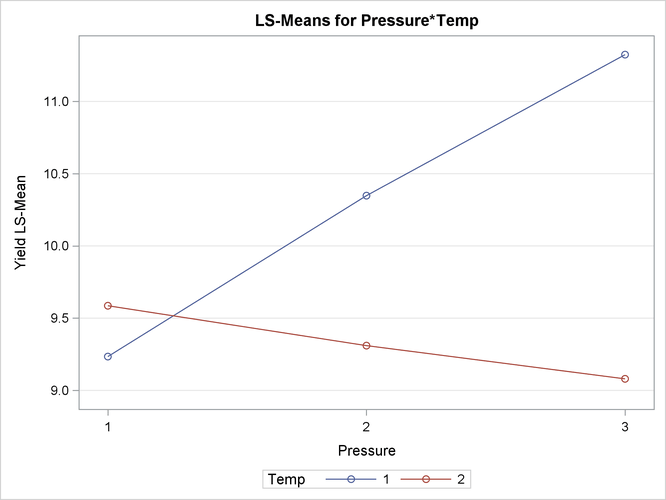Figure 45.26: Pressure Comparisons at a Given Temperature

The GLIMMIX Procedure

Tests of Effect Slices for Pressure*Temp
Sliced By Temp
Temp Num DF Den DF F Value Pr > F
1 2 20 4.95 0.0179
2 2 20 0.29 0.7508

Simple Effect Comparisons of Pressure*Temp Least Squares Means By Temp
Simple Effect
Level
Pressure _Pressure Estimate Standard
Error
DF t Value Pr > |t|
Temp 1 1 2 -1.1140 0.6645 20 -1.68 0.1092
Temp 1 1 3 -2.0900 0.6645 20 -3.15 0.0051
Temp 1 2 3 -0.9760 0.6645 20 -1.47 0.1575
Temp 2 1 2 0.2760 0.6645 20 0.42 0.6823
Temp 2 1 3 0.5060 0.6645 20 0.76 0.4553
Temp 2 2 3 0.2300 0.6645 20 0.35 0.7329

The slope differences are evident by the change in sign for comparisons within temperature 1 and within temperature 2. There is a significant effect of pressure at temperature 1 (p = 0.0179), but not at temperature 2 (p = 0.7508).

##### Pairwise Difference Plot (Diffogram)

Graphical displays of LS-means-related analyses consist of plots of all pairwise differences (DiffPlot), plots of differences against a control level (ControlPlot), and plots of differences against an overall average (AnomPlot). The following data set is from an experiment to investigate how snapdragons grow in various soils (Stenstrom 1940). To eliminate the effect of local fertility variations, the experiment is run in blocks, with each soil type sampled in each block. See the "Examples" section of Chapter 46: The GLM Procedure, for an in-depth analysis of these data.

data plants;
input Type $@; do Block = 1 to 3; input StemLength @; output; end; datalines; Clarion 32.7 32.3 31.5 Clinton 32.1 29.7 29.1 Knox 35.7 35.9 33.1 ONeill 36.0 34.2 31.2 Compost 31.8 28.0 29.2 Wabash 38.2 37.8 31.9 Webster 32.5 31.1 29.7 ;  The following statements perform the analysis of the experiment with the GLIMMIX procedure: ods graphics on; ods select LSMeans DiffPlot; proc glimmix data=plants order=data plots=Diffogram; class Block Type; model StemLength = Block Type; lsmeans Type; run; ods graphics off;  The PLOTS= option in the PROC GLIMMIX statement requests that plots of pairwise least squares means differences are produced for effects that are listed in corresponding LSMEANS statements. This is the Type effect. The Type LS-means are shown in Figure 45.27. Note that the order in which the levels appear corresponds to the order in which they were read from the data set. This was accomplished with the ORDER= DATA option in the PROC GLIMMIX statement. Figure 45.27: Least Squares Means for Type Effect The GLIMMIX Procedure Type Least Squares Means Type Estimate Standard Error DF t Value Pr > |t| Clarion 32.1667 0.7405 12 43.44 <.0001 Clinton 30.3000 0.7405 12 40.92 <.0001 Knox 34.9000 0.7405 12 47.13 <.0001 ONeill 33.8000 0.7405 12 45.64 <.0001 Compost 29.6667 0.7405 12 40.06 <.0001 Wabash 35.9667 0.7405 12 48.57 <.0001 Webster 31.1000 0.7405 12 42.00 <.0001 Because there are seven levels of Type in this analysis, there are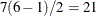pairwise comparisons among the least squares means. The comparisons are performed in the following fashion: the first level of Type is compared against levels 2 through 7; the second level of Type is compared against levels 3 through 7; and so forth. The default difference plot for these data is shown in Figure 45.28. The display is also known as a "mean-mean scatter plot" (Hsu 1996; Hsu and Peruggia 1994). It contains 21 lines rotated by 45 degrees counterclockwise, and a reference line (dashed 45-degree line). The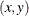coordinate for the center of each line corresponds to the two least squares means being compared. Suppose that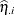and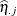denote the ith and jth least squares mean, respectively, for the effect in question, where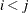according to the ordering of the effect levels. If the ABS option is in effect, which is the default, the line segment is centered at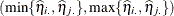. Take, for example, the comparison of "Clarion" and "Compost" types. The respective estimates of their LS-means are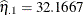and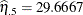. The center of the line segment for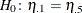is placed at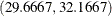. The length of the line segment for the comparison between means i and j corresponds to the width of the confidence interval for the difference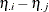. This length is adjusted for the rotation in the plot. As a consequence, comparisons whose confidence interval covers zero cross the 45-degree reference line. These are the nonsignificant comparisons. Lines associated with significant comparisons do not touch or cross the reference line. Because these data are balanced, the estimated standard errors of all pairwise comparisons are identical, and the widths of the line segments are the same. Figure 45.28: LS-Means Plot of Pairwise Differences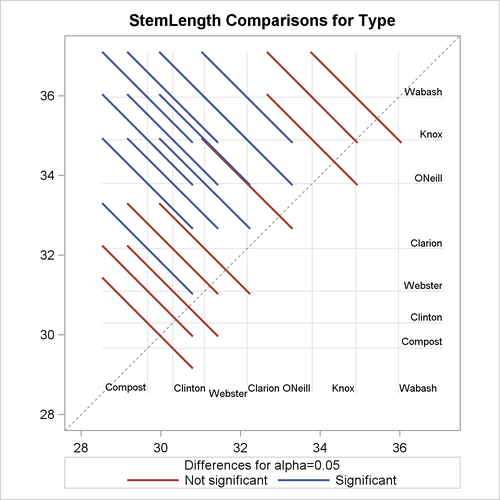The background grid of the difference plot is drawn at the values of the least squares means for the seven type levels. These grid lines are used to find a particular comparison by intersection. Also, the labels of the grid lines indicate the ordering of the least squares means. In the next set of statements, the NOABS and CENTER suboptions of the PLOTS= DIFFOGRAM option in the LSMEANS statement modify the appearance of the diffogram: ods graphics on; proc glimmix data=plants order=data; class Block Type; model StemLength = Block Type; lsmeans Type / plots=diffogram(noabs center); run; ods graphics off;  The NOABS suboption of the difference plot changes the way in which the GLIMMIX procedure places the line segments (Figure 45.29). If the NOABS suboption is in effect, the line segment is centered at the point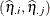,. For example, the center of the line segment for a comparison of "Clarion" and "Compost" types is centered at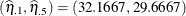. Whether a line segment appears above or below the reference line depends on the magnitude of the least squares means and the order of their appearance in the "Least Squares Means" table. The CENTER suboption places a marker at the intersection of the least squares means. Because the ABS option places lines on the same side of the 45-degree reference, it can help to visually discover groups of significant and nonsignificant differences. On the other hand, when the number of levels in the effect is large, the display can get crowded. The NOABS option can then provide a more accessible resolution. Figure 45.29: Diffogram with NOABS and CENTER Options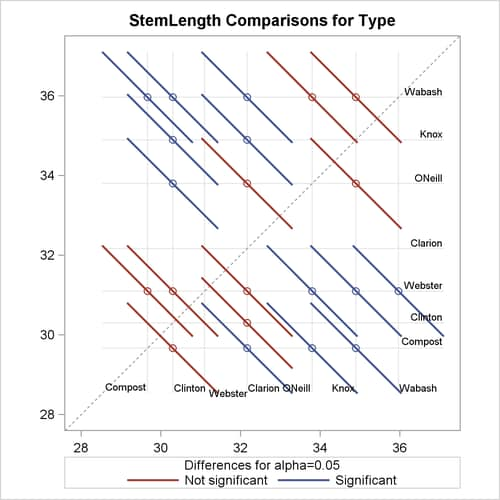##### Least Squares Mean Control Plot The following SAS statements create the same data set as before, except that one observation for Type="Knox" has been removed for illustrative purposes: data plants; input Type$ @;
do Block = 1 to 3;
input StemLength @;
output;
end;
datalines;
Clarion   32.7 32.3 31.5
Clinton   32.1 29.7 29.1
Knox      35.7 35.9   .
ONeill    36.0 34.2 31.2
Compost   31.8 28.0 29.2
Wabash    38.2 37.8 31.9
Webster   32.5 31.1 29.7
;


The following statements request control plots for effects in LSMEANS statements with compatible option:

ods graphics on;
ods select Diffs ControlPlot;

proc glimmix data=plants order=data plots=ControlPlot;
class Block Type;
model StemLength = Block Type;
lsmeans Type / diff=control('Clarion') adjust=dunnett;
run;

ods graphics off;


The LSMEANS statement for the Type effect is compatible; it requests comparisons of Type levels against "Clarion," adjusted for multiplicity with Dunnett’s method. Because "Clarion" is the first level of the effect, the LSMEANS statement is equivalent to

lsmeans type / diff=control adjust=dunnett;


The "Differences of Type Least Squares Means" table in Figure 45.30 shows the six comparisons between Type levels and the control level.

Figure 45.30: Least Squares Means Differences

The GLIMMIX Procedure

Differences of Type Least Squares Means
Adjustment for Multiple Comparisons: Dunnett
Type _Type Estimate Standard Error DF t Value Pr > |t| Adj P
Clinton Clarion -1.8667 1.0937 11 -1.71 0.1159 0.3936
Knox Clarion 2.7667 1.2430 11 2.23 0.0479 0.1854
ONeill Clarion 1.6333 1.0937 11 1.49 0.1635 0.5144
Compost Clarion -2.5000 1.0937 11 -2.29 0.0431 0.1688
Wabash Clarion 3.8000 1.0937 11 3.47 0.0052 0.0236
Webster Clarion -1.0667 1.0937 11 -0.98 0.3504 0.8359

The two rightmost columns of the table give the unadjusted and multiplicity-adjusted p-values. At the 5% significance level, both "Knox" and "Wabash" differ significantly from "Clarion" according to the unadjusted tests. After adjusting for multiplicity, only "Wabash" has a least squares mean significantly different from the control mean. Note that the standard error for the comparison involving "Knox" is larger than that for other comparisons because of the reduced sample size for that soil type.

In the plot of control differences a horizontal line is drawn at the value of the "Clarion" least squares mean. Vertical lines emanating from this reference line terminate in the least squares means for the other levels (Figure 45.31).

The dashed upper and lower horizontal reference lines are the upper and lower decision limits for tests against the control level. If a vertical line crosses the upper or lower decision limit, the corresponding least squares mean is significantly different from the LS-mean in the control group. If the data had been balanced, the UDL and LDL would be straight lines, because all estimates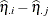would have had the same standard error. The limits for the comparison between "Knox" and "Clarion" are wider than for other comparisons, because of the reduced sample size for the "Knox" soil type.

Figure 45.31: LS-Means Plot of Differences against a Control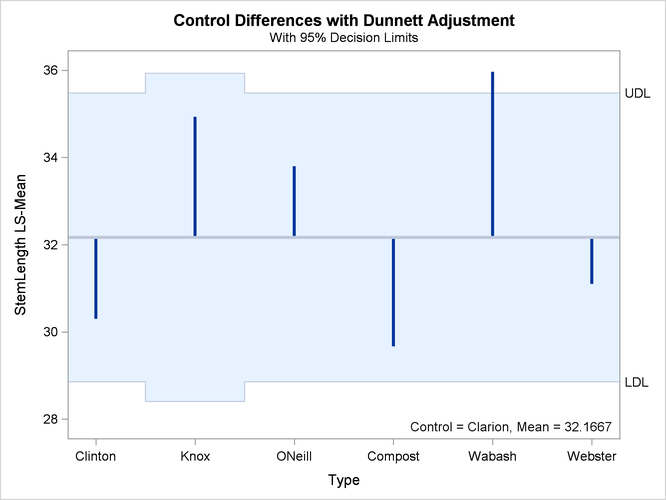The significance level of the decision limits is determined from the ALPHA= level in the LSMEANS statement. The default are 95% limits. If you choose one-sided comparisons with DIFF= CONTROLL or DIFF= CONTROLU in the LSMEANS statement, only one of the decision limits is drawn.

##### Analysis of Means (ANOM) Plot

The analysis of means in PROC GLIMMIX compares least squares means not by contrasting them against each other as with all pairwise differences or control differences. Instead, the least squares means are compared against an average value. Consequently, there are k comparisons for a factor with k levels. The following statements request ANOM differences for the Type least squares means (Figure 45.32) and plots the differences (Figure 45.33):

ods graphics on;
ods select Diffs AnomPlot;
proc glimmix data=plants order=data plots=AnomPlot;
class Block Type;
model StemLength = Block Type;
lsmeans Type / diff=anom;
run;
ods graphics off;


Figure 45.32: ANOM LS-Mean Differences

The GLIMMIX Procedure

Differences of Type Least Squares Means
Type _Type Estimate Standard Error DF t Value Pr > |t|
Clarion Avg -0.2635 0.7127 11 -0.37 0.7186
Clinton Avg -2.1302 0.7127 11 -2.99 0.0123
Knox Avg 2.5032 0.9256 11 2.70 0.0205
ONeill Avg 1.3698 0.7127 11 1.92 0.0809
Compost Avg -2.7635 0.7127 11 -3.88 0.0026
Wabash Avg 3.5365 0.7127 11 4.96 0.0004
Webster Avg -1.3302 0.7127 11 -1.87 0.0888

At the 5% level, the "Clarion," "O’Neill," and "Webster" soil types are not significantly different from the average. Note that the artificial lack of balance introduced previously reduces the precision of the ANOM comparison for the "Knox" soil type.

Figure 45.33: LS-Means Analysis of Means (ANOM) Plot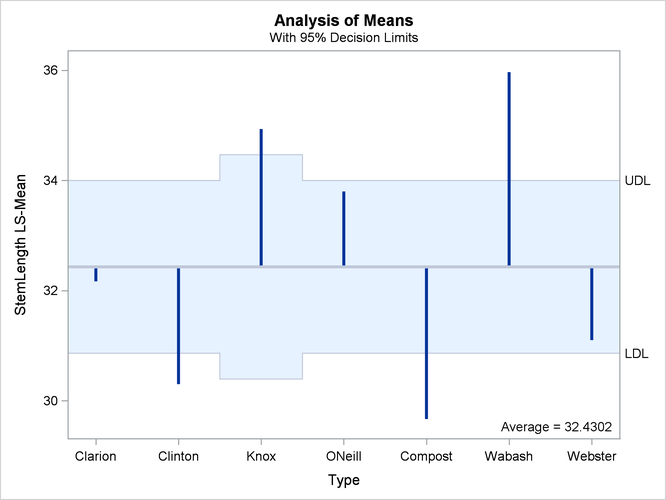The reference line in the ANOM plot is drawn at the average. Vertical lines extend from this reference line upward or downward, depending on the magnitude of the least squares means compared to the reference value. This enables you to quickly see which levels perform above and below the average. The horizontal reference lines are 95% upper and lower decision limits. If a vertical line crosses the limits, you conclude that the least squares mean is significantly different (at the 5% significance level) from the average. You can adjust the comparisons for multiplicity by adding the ADJUST= NELSON option in the LSMEANS statement.